Contents >> Applied Mathematics >> Matrix Algebra >> Principles of Matrix Calculation >> The inverse matrix

 Matrix Algebra - The inverse matrix The inverse matrix The matrix which at multiplication both on the right and at the left on the given matrix gives an identity matrix is called an inverse matrix. Let's designate an inverse matrix to a matrix A as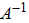, then according to the definition we’ll receive: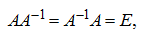where E – an identity matrix. The square matrix is called regular ( non- singular ) if its determinant is not equal to zero. Otherwise, it is called singular . The theorem takes place: any regular matrix has an inverse matrix . Operation of an inverse matrix finding is called the inversion of a matrix. We’ll consider algorithm of the inversion of a matrix. Let the regular n -th order matrix is given: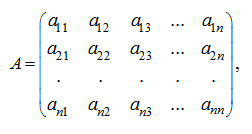where  Δ = det A ≠ 0. Algebraic addition of an element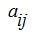of the n -th order matrix A is called the determinant of the ( n –1)-th order matrix taken with a certain sign and received by deletion of the i -th row and the j -th column of the matrix A :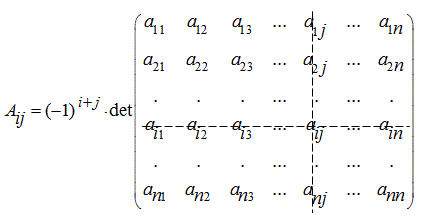Let’s make the so-called adjoint matrix: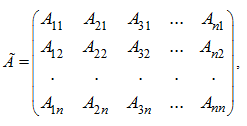where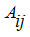– algebraic additions of appropriating elementsof matrix A . Let's notice, that algebraic additions of elements of the matrix A rows are placed in appropriating columns of the matrix Ã , that is a matrix transposing is made at the same time. Dividing all elements of matrix Ã by Δ – value of determinant of a matrix A , we’ll receive as a result an inverse matrix: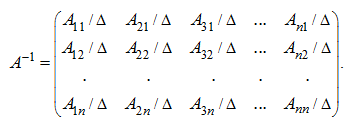Let's note a number of special properties of an inverse matrix: 1) for the given matrix A its inverse matrixis unique; 2) if there is an inverse matrixa right and a left inverse matrices coincide with it; 3) the singular square matrix has no an inverse matrix. The basic properties of an inverse matrix: 1) the determinant of an inverse matrix and a determinant of an initial matrix are inverses; 2) the inverse matrix of product of square matrices is equal to product of inverse matrices of the factors, taken upside-down: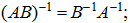3) the transposed inverse matrix is equal to an inverse matrix of the given transposed matrix: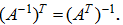E x a m p l e .  Calculate a matrix, inverse to the given one: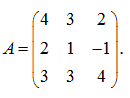S o l u t i o n . The determinant of the matrix A is equal: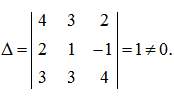Consequently, the matrix A is regular. The adjoint matrix Ã looks like: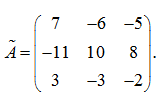Dividing all elements of the adjoint matrix Ã by Δ = 1, we’ll receive the inverse matrix: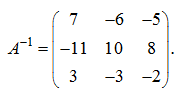Let's check up, that the quality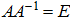is valid: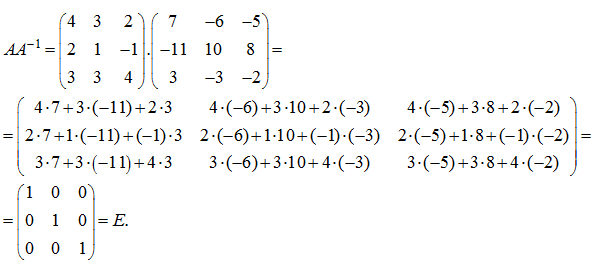Thus, the found matrixis inverse for the given matrix A .

 < Previous Contents Next >